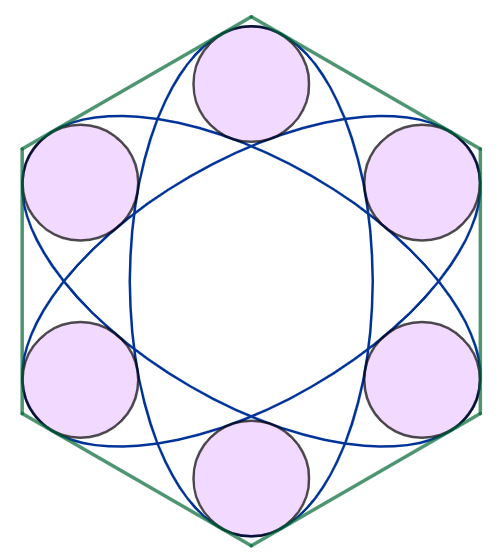# Absolutely Atomic Arrangement!

Geometry Level 5As shown above, three identical ellipses of the minor axis $2$ are positioned, such that each of them has four points of tangency with the regular hexagon at two opposite sides. There exists the unique value of eccentricity, such that all six identical circles has one point of tangency with each of the three ellipses.

If the side length of the hexagon can be expressed as

$\sqrt{\dfrac{A}{B}\left(C + D\sqrt{E}\cos\left(\dfrac{F}{G}\pi\right)\right)}$

where

• $A,B,C,D,E,F,G$ are positive integers
• $\gcd(A,B) = \gcd(C,D) = \gcd(F,G) = 1$
• $E$ is square-free
• $0 < 2F < G$

Input $A + B + C + D + E + F + G$ as your answer.

×